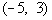Krishna
0

Step 1: Group all the algebraic terms together on one side and group all the numeric terms together on the other side of the inequality.

Step 2: Recall the rules of manipulating Inequalities to simplify inequalities

Rule 1: Adding or subtracting the same quantity from both sides of an  inequality leaves the inequality symbol unchanged.

Rule 2: Multiplying or dividing by a positive number leaves the inequality symbol unchanged.

Rule 3:  Multiplying or dividing by a negative number reverses the inequality sign.

EXAMPLE: > changes to <, and vice versa.

Rule 4: Inverting fractions reverses the inequality sign.

EXAMPLE: \frac{2}{5}\ <\ \frac{2}{3}\ =\ \frac{5}{2}\ >\ \frac{3}{2}

Step 3: Write the quadratic inequality in standard form.

Step 4: Solve the quadratic equation ax^2+bx+c, by factoring to get the boundary point(s).

EXAMPLE: x^2 + 2x - 15 = 0

(x+5)(x-3) = 0

x = -5 or x = 3

Step 5: Use the boundary points found in step 4 to mark off test intervals on the number line and list all of the factors found in step 4.

EXAMPLE: Note that the two boundary points create three sections on the graph:  (-\infty, -5), (-5, 3), (3, \infty).

Step 6 : Substitute the numbers in the interval on the quadratic equation, and make sure is it satisfying the inequality or not

EXAMPLE: If we chose a number in the first interval, (-\infty, -5), like -6 (I could have used -10, -25, or -10000 as long as it is in the interval), it would make both factors negative:

x + 5 = 0    and  x - 3 =0

-6 + 5 = -1 and -6 - 3 = -9

(-1)(-9) < 0

9 <  0 not satisfied,

take the another interval and verify

If we chose a number in the second interval,, like 0 (I could have used -4, -1, or 2 as long as it is in the interval), it would make x + 5 positive and x - 3 negative:

0 + 5 = 5 and 0 - 3 = -3

(5)(-3) < 0

-8 < 0 satisfying

Step 7: Take the interval which satisfies the inequality equation and find out the suitable Interval notation

NOTE: Open interval indicating all values between -5 and 3

Closed intervals indicating all values less than or equal to -5 or greater than or equal to 3﻿